## Screenshots

•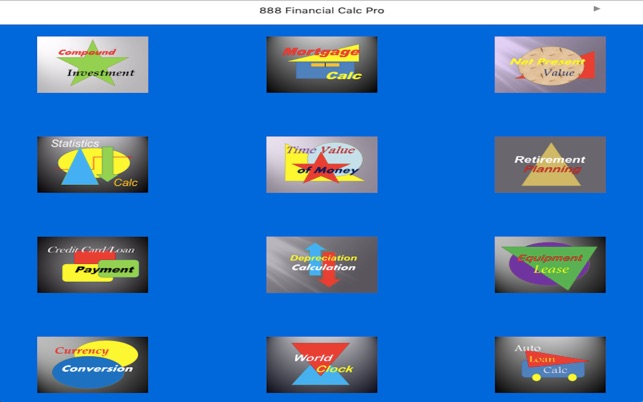•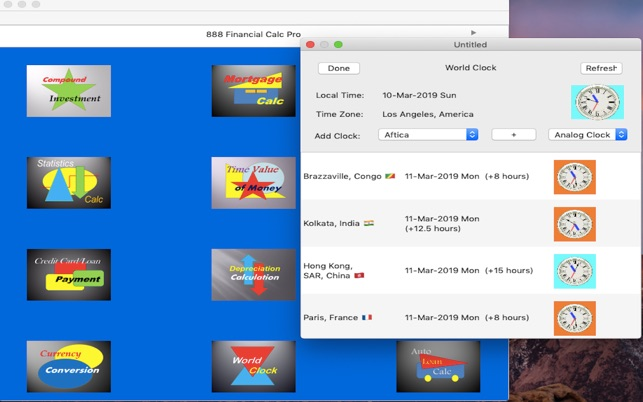•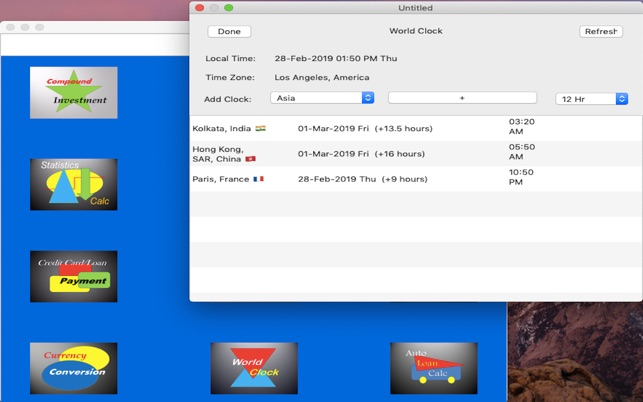•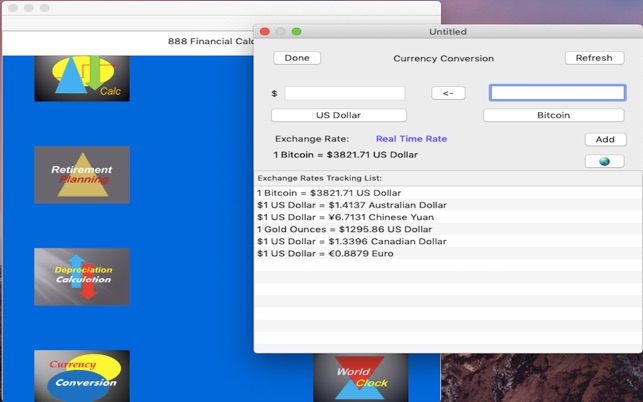•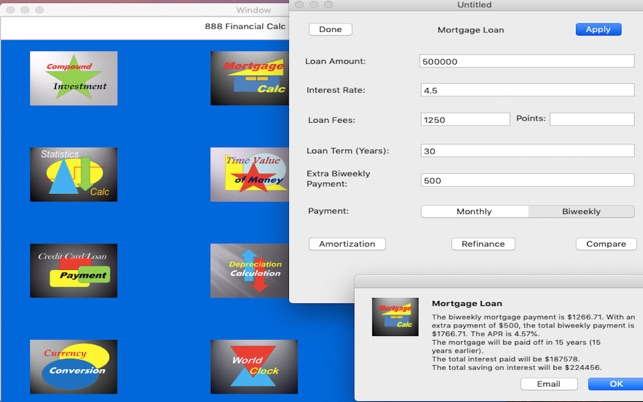•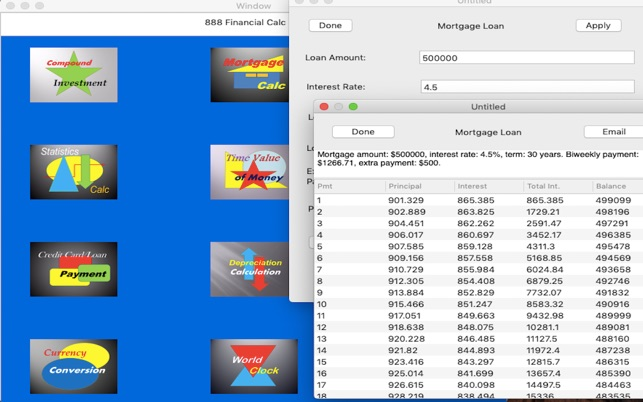## Description

This advanced financial calculator app is designed for financial planners and ordinary users to aid their daily financial planning/calculations (TVM, NPV, IRR, loans, tips) and international investments/travels.

Specifically, the app contains:
1. Real-time and historical currency exchange rate trackers/converters:
(a) Supports over 160 currencies, precious metals
and Bitcoin.
(b) Real time mode and off-line mode.
(c) User-defined currency exchange tracking list.
(d) Currency symbols are shown with rates.
(e) Historical currency exchange rates.
(f) Email historical exchange rate changes in
CSV files.
2. World Clock:
(a) User-defined list of countries to track.
(b) Auto refresh of clocks every second.
(c) Clocks with day light saving time are annotated.
(d) 12hr/24hr digital time clock display option.
(e) Analog clock display option.
(f) Country flags are displayed in tracking list.
3. Time Value of Money Calculator:
Calculate present value, future value, annuity
(ordinary or due), annual interest rate or term of an
investment.
4. Net Present Value and Internal Rate of Return Calc
Calculate the NPV and/or IRR of an investment.
5. Tip Calculator:
(a) Calculate tips, sales taxes and split bill payments.
(b) Calculate total bill by allowing users to enter
individual bill item's amount into a table.
6. Auto Loan Calculator:
(a) Calculate monthly auto loan payment, term
or the maximum affordable auto price.
(b) Option to specify trade-in value is taxable or not.
(c) Option to include or exclude sales tax in loan.
(d) Amortization table.
7. Mortgage Loan Calculator:
(a) Calculate mortgage payment and interest APR.
(b) Calculate monthly or biweekly payment amount.
(c) Amortization table.
(d) Allow users to compare two mortgages programs
in terms of monthly payments, total interests paid
and loan terms.
(e) Allow users to determine how much money and
time they may save by refinancing their mortgage.
8. Credit Card or Loan Payment Calculator:
(a) Calculate personal/commercial loan payment,
term or maximum loan amount that can be
borrowed.
(b) Calculate credit card monthly payment, term, or
maximum balance that can be charged.
(c) Simple interest and various compound interest
options.
(d) User-specified number of payments and payment
frequency.
9. Compound Interest Investment:
Calculate the total interest that will be accumulated
given an investment amount, interest rate and the
investment term.
10. Bond Investment Calculator:
Calculate Yield to Maturity, bond duration and
Yield to Call.
11. Retirement Planning Calculator:
Calculate either the annual amount needed to be
saved to reach a retirement goal, or the amount will
be saved when reaching retirement and how many
years it will last.
12. Depreciation calculation and depreciation table:
(a) Calculate an asset's straight-line, declining-
balance or sum-of-the-years-digits depreciation.
(b) Depreciation table.
13. Equipment Lease Calculator:
Calculate either the monthly payment of the annual
yield rate of a lease for an asset.
14. Unit conversion:
Convert temperature, weight, distance, and liquid
units.
15. Statistics Calculator:
Calculate the mean, weighted mean, median,
standard deviation/variance or linear estimation for
any set of user data.

## What’s New

Version 1.4

Fixed on Mac Book Pro, analog clocks disappeared from the world clock tracking list, after a city is deleted from the list.

## Ratings and Reviews

jkwCFP ,

### Love it!!

I like 888 Financial Calculator because it integrates present value, time value, compound rate, retirement calculation, unit conversion, currency conversion, world clock and more into one app. It is practical and user friendly. I rate this app higher than many other financial calculator apps because it brings many useful calculations into one easy to use app.

## Information

Seller
Dynetix Design Solutions Inc
Size
6.3 MB
Category
Finance
Compatibility

OS X 10.11 or later, 64-bit processor

Languages

English

Age Rating
Rated 4+
•